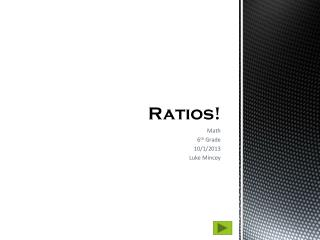DownloadDownload PresentationRatios!

# Ratios!

Télécharger la présentation## Ratios!

- - - - - - - - - - - - - - - - - - - - - - - - - - - E N D - - - - - - - - - - - - - - - - - - - - - - - - - - -
##### Presentation Transcript

1. Ratios! Math 6th Grade 10/1/2013 Luke Mincey

2. Main Menu Content Assessment CCSS.Math.Content.6.RP.A.1 Understand the concept of a ratio and use ratio language to describe a ratio relationship between two quantities. References End Show

3. What is a ratio? • A “Ratio” represents how much of one thing there is compared to another thing. • For instance: Mr. Smith has a motorcycle and a car. We can compare the number of car wheels to the number of motorcycle wheels Mr. Smith has with a ratio. Content 1.1 Ratios!

4. How do we write a ratio? • We can write a ratio three ways; word notation, odds notation, and fractional notation. • The ratio of “car wheels” to “motorcycle wheels” that Mr. Smith has is represented like this: • Word notation – four to two • Odds notation - 4:2 • Fractional notation – 4/2 • It is important to note that “car” came before “motorcyle.” • Whichever word comes first; it’s number must come first in the ratio. Content 1.2

5. Using Ratios • A ratio can be scaled up • Here we have the ratio of three to one (3:1 or 3/1) • And we scale it up to sixto two (6:2 or 6/2) Content 1.3

6. Using Ratios (Cont’d) • When scaling up we must be sure to multiply both numbers with the same value. • For example; If Mr. Smith purchased two more cars and two more motorcycles we can scale up the ratio of, “tires on a car” to “tires on a motorcycle,” to the ratio of “tires on three cars” to “tires on three motorcycles” which would look like this: • 4*3:2*3  12:6 • Notice that we multiple each number in the original ratio by the same value, 3. • Mr. Smith still has the same ratio of tires. Content 1.4

7. “Part-to-part” and “Part-to-whole” ratios • The example ratio of “car” to “motorcycle tires (4:2) is a “part-to-part” ratio. • A “part-to-whole” ratio is a ratio that compares a part to a whole lot. • For example; the “part-to-whole” ratio for number of motorcycle tires Mr. Smith owns to the total number of tires Mr. Smith owns is 2:6 • Likewise; the “part-to-whole” ratio for number of car tires Mr. Smith owns to the total number of tires Mr. Smith owns is 4:6 • For more on ratios, visit these sites: • http://www.aaamath.com/rat62ax2.htm • http://www.purplemath.com/modules/ratio.htm Content 1.5

8. How can we write a ratio? • Word Notation • Fraction Notation • Odds Notation • All of the above Exercise 1

9. There are 20 students in Mr. Bill’s class. 12 students are male. 8 Students are female. What is the “part-to-whole” ratio of male students in Mr. Bill’s class? • 12:8 • 12:20 • 8:20 • 12/8 Exercise 2

10. Ashley has 6 pencils and 4 pens. The ratio of Ashley’s pens to Ashley’s pencils is 4:6. If Ashley scales up her ratio of pens to pencils by three, how many pens will Ashley have? • 6 pens • 12 pens • 16 pens • 4 pens Exercise 3

11. Last basketball game, Tyler took a total of 17 shots. Out of the 17 shots, Tyler made 6. What is the ratio of shots Tyler made to shots Tyler missed. • 6:17 • 17:11 • 6:11 • 11:6 Exercise 4

12. In a bag of M&M’s you count 12 blue M&M’s and 18 red M&M’s. What is the ratio of red to blue M&M’s in fractional notation? • 18/12 • 12:18 • 18:12 • 12 to 18 Exercise 5

13. Correct! Well done!

14. Incorrect, try again!

15. Pierce, Rod. "Ratios" Math Is Fun. Ed. Rod Pierce. 27 Aug 2013. 1 Oct 2013 <http://www.mathsisfun.com/numbers/ratio.html> References